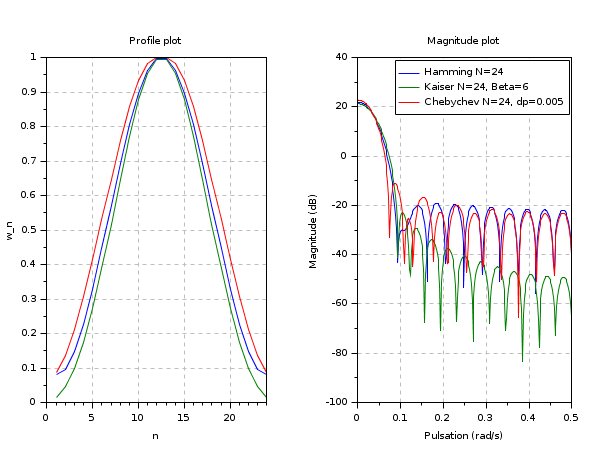Scilab Home page | Wiki | Bug tracker | Forge | Mailing list archives | ATOMS | File exchange
Change language to: Français - Português - 日本語 - Русский

Please note that the recommended version of Scilab is 6.1.1. This page might be outdated.
See the recommended documentation of this function

Scilab Help >> Signal Processing > filters > window

# window

compute symmetric window of various type

### Calling Sequence

win_l=window('re',n)
win_l=window('tr',n)
win_l=window('hn',n)
win_l=window('hm',n)
win_l=window('kr',n,Beta)
[win_l,cwp]=window('ch',n,par)

### Arguments

n

window length

par

parameter 2-vector par=[dp,df]), where dp (0<dp<.5) rules the main lobe width and df rules the side lobe height (df>0).

Only one of these two value should be specified, the other one must be equal to a nonpositive value.

Beta

Kaiser window parameter Beta >0).

win

window

cwp

unspecified Chebyshev window parameter

### Description

function which calculates various symmetric window for Digital signal processing.

• The Kaiser window is a nearly optimal window function. Beta is an arbitrary positive real number that determines the shape of the window, and the integer n is the length of the window.

By construction, this function peaks at unity for k = n/2 , i.e. at the center of the window, and decays exponentially towards the window edges. The larger the value of Beta, the narrower the window becomes; Beta = 0 corresponds to a rectangular window. Conversely, for larger Beta the width of the main lobe increases in the Fourier transform, while the side lobes decrease in amplitude. Thus, this parameter controls the tradeoff between main-lobe width and side-lobe area.

 Beta window shape 0 Rectangular shape 5 Similar to the Hamming window 6 Similar to the Hann window 8.6 Similar to the Blackman window
• The Chebyshev window minimizes the mainlobe width, given a particular sidelobe height. It is characterized by an equiripple behavior, that is, its sidelobes all have the same height.

• The Hann and Hamming windows are quite similar, they only differ in the choice of one parameter alpha: w=alpha+(1 - alpha)*cos(2*%pi*x/(n-1)) alpha is equal to 1/2 in Hann window and to 0.54 in Hamming window.

### Examples

clf()
N = 24;
whm = window('hm', N); // Hamming window
wkr = window('kr', N, 6); // Hamming Kaiser window
wch = window('ch', N, [0.005 -1]); // Chebychev window

// Plot the window profile
subplot(121);
plot((1:N)', [whm; wkr; wch]')
set(gca(), 'grid', [1 1]*color('gray'))
xlabel("n")
ylabel("w_n")
title(gettext("Profile plot"))

// Plot the magnitude of the frequency responses
n = 256;
[Whm,fr] = frmag(whm, n);
[Wkr,fr] = frmag(wkr, n);
[Wch,fr] = frmag(wch, n);
subplot(122);
plot(fr', 20*log10([Whm; Wkr; Wch]'))
set(gca(), 'grid', [1 1]*color('gray'))
ylabel(gettext("Magnitude (dB)"))
legend(["Hamming N=24"; "Kaiser N=24, Beta=6"; "Chebychev N=24, dp=0.005"]);
title(gettext("Magnitude plot"))clf()
N = 140;
w1 = window('kr', N, 1);
w2 = window('kr', N, 2);
w4 = window('kr', N, 4);
w8 = window('kr', N, 8);
w16 = window('kr', N, 16);

// Plot the window profile
plot((1:N)', [w1; w2; w4; w8; w16]')
set(gca(), 'grid', [1 1]*color('gray'))
legend("$\beta = "+string([1;2;4;8;16])+'$',[55,0.3])
xlabel("n")
ylabel("w_n")
title(gettext("Comparison of Kaiser window profiles"))clf()
N = 140;
w1 = window('ch', N, [0.001 -1]);
w2 = window('ch', N, [0.05 -1]);
w3 = window('ch', N, [-1 0.4]);

// Plot the window profile
subplot(121);
plot((1:N)', [w1; w2; w3]')
set(gca(), 'grid', [1 1]*color('gray'))
//legend("$\beta = "+string([1;2;4;8;16])+'$',[55,0.3])
xlabel("n")
ylabel("w_n")
title(gettext("Comparison of Chebychev window profiles"))

// Plot the magnitude of the frequency responses
n = 256;
[W1,fr] = frmag(w1, n);
[W2,fr] = frmag(w2, n);
[W3,fr] = frmag(w3, n);
subplot(122);
plot(fr', 20*log10([W1; W2; W3]'))
set(gca(), 'grid', [1 1]*color('gray'))
ylabel(gettext("Magnitude (dB)"))
legend(["Chebychef dp=0.001"; "Chebychef dp=0.05"; "Chebychef df=0.4"]);
title(gettext("Chebychev window Magnitude plot"))• wfir — linear-phase FIR filters
• frmag — magnitude of FIR and IIR filters
• ffilt — coefficients of FIR low-pass

### Bibliography

IEEE. Programs for Digital Signal Processing. IEEE Press. New York: John Wiley and Sons, 1979. Program 5.2.